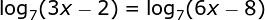# Exponentials and Logarithms Chapter Exam

Free Practice Test Instructions:

Choose your answer to the question and click 'Continue' to see how you did. Then click 'Next Question' to answer the next question. When you have completed the free practice test, click 'View Results' to see your results. Good luck!

#### Question 3 3. Evaluate the following: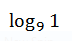#### Question 5 5. Which value of x will make the equation below true?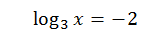#### Question 7 7. Solve for x: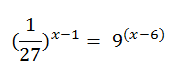#### Question 10 10. What is the base of this log?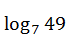#### Question 11 11. Evaluate the following: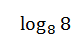#### Question 12 12. Write the following expression as a single logarithm. Simplify as much as possible.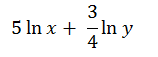#### Question 13 13. Use the properties of logarithmic expressions to undo the following expression. Which of the following expressions is it equal to? (hint: ln(e) = 1)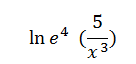#### Question 14 14. Which of the following is equal to?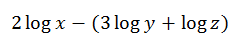#### Question 15 15. Solve the following equation for x.